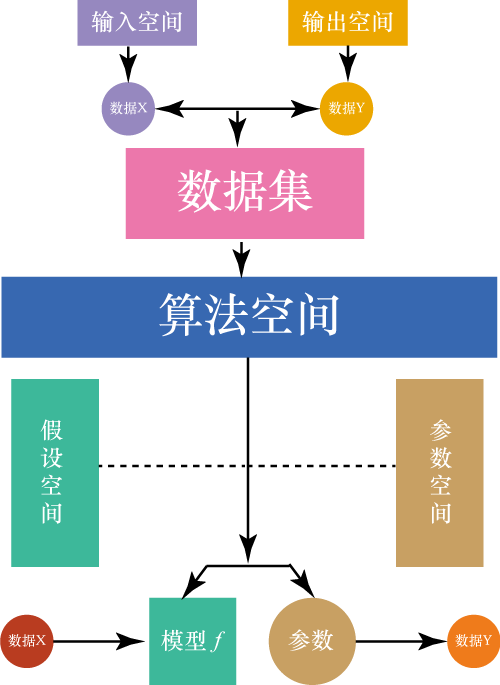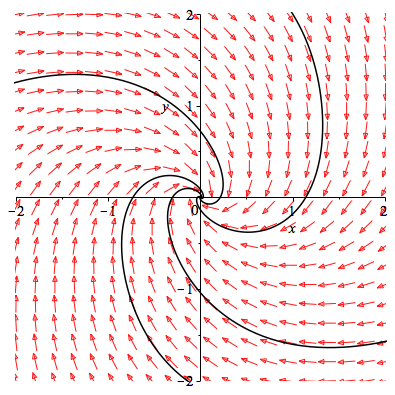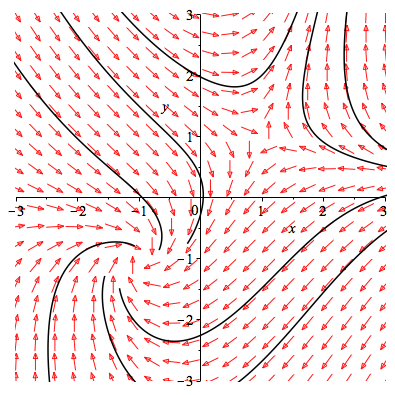### 一、机器学习若干符号解释

#### 3、假设空间：

##### 1、如果真实的世界的关系是 $\displaystyle y=h(\boldsymbol{x})$， 世界充满噪声。所以 $\displaystyle y=h(\boldsymbol{x})+e$。

1、世界是这样的： $\displaystyle p(y=h(\boldsymbol{x})\mid\boldsymbol{x})$
2、我们观察到的世界是这样的：$\displaystyle \mathcal{D}=\{(\boldsymbol{x}_i,y_i)\}_{i=1}^{n}$
3、我们假设世界是这样的： $\displaystyle p(y=f(\boldsymbol{x)\mid }\boldsymbol{x},\mathcal{D},M)$[^1]，其中 $\displaystyle M$是模型(算法)。

$\displaystyle \varepsilon=y-f=y-h+h-f=y-h+h-\mathrm{E}[f]+\mathrm{E}[f]-f$
$\displaystyle \mathrm{E}[\varepsilon^2]=\mathrm{Var}[e]+\left(h-\mathrm{E}[f]\right)^2+\mathrm{E}\left[\left(f-\mathrm{E}[f]\right)^2\right]$
$$平方损失期望=噪声方差+偏误^2+模型方差$$

4、我们还可以写成：
$\displaystyle \mathcal{H} =\{f\mid p(y=f(\boldsymbol{x})\mid\boldsymbol{x}, \mathcal{D})\}=\{f(\boldsymbol{\beta})\mid p(y=f(\boldsymbol{x};\boldsymbol{\beta})\mid \boldsymbol{x},\mathcal{D};\boldsymbol{\beta}),\boldsymbol{\beta}\in \mathbb{R}^k\}$。这里的$\displaystyle \mathcal{H}$是模型 $f$的集合。

##### 2、这里的符号有一个重要的解释：

$\displaystyle y$是一个随机变量，它的取值是 $\displaystyle y=y_i$。 $\displaystyle \boldsymbol{x}$表示的是 $\displaystyle n$个 $\displaystyle k$维输入数据。也就是说 $\displaystyle \boldsymbol{x}$也是一个变量，不过是向量的形式。它的取值是 $\displaystyle \boldsymbol{x}=\boldsymbol{x}_i$。

##### 3、换一个程序员比较好理解的说法：

$\displaystyle y,\boldsymbol{x}$是一个类。而 $\displaystyle y_i,\boldsymbol{x}_i$是一个实例。所以一个实例 $\displaystyle P(y_i\mid \boldsymbol{x}_i,\mathcal{D})$，又有 $\displaystyle P(y_{n+1}\mid \boldsymbol{x}_{n+1},\mathcal{D})$是一个数或者一个概率。$\displaystyle \hat{y},\hat{y}_i$也是类和实例的区别。

#### 4、算法空间

$\displaystyle \zeta\in\mathcal{L}$，它是算法的集合。

#### 5、参数空间

$\displaystyle \boldsymbol{\beta} \in\mathbb{R}^k$。这里的元素我们将 $\displaystyle \mathbb{R}^k$的k维有序组与向量矩阵$\mathop{\boldsymbol{\beta}}\limits_{(k\times 1)}$等同，以方便表达。

#### 6、概念总结#### 7、指示函数，或者叫示性函数

$\displaystyle \mathrm{I}_x(A)=\begin{cases}1&\text{if }x\in A\\0&\text{if }x\notin A\end{cases}$

### 二、回归模型

#### 1、线性回归模型：

$y_i=\boldsymbol{x}_{i}^T\boldsymbol{\beta}+\epsilon_i=\boldsymbol{x}_{i,:\,}^T\boldsymbol{\beta}+\epsilon_i$
$\boldsymbol{y}=\boldsymbol{X}\boldsymbol{\beta}+\boldsymbol{\epsilon}$
$S=\boldsymbol{\epsilon}^{\text{T}}\boldsymbol{\epsilon}$

$$\displaystyle \mathop{\boldsymbol{y}}\limits_{(n\times 1)}=\underbrace{\mathop{\boldsymbol{X}}\limits_{(n\times k)} \mathop{\boldsymbol{\beta}}\limits_{(k\times 1)}}_{n\times k} +\mathop{\boldsymbol{\epsilon}}\limits_{(n\times 1)}$$

#### 2、梯度下降算法：$\boldsymbol{\beta}: =\boldsymbol{\beta}-\alpha\nabla S$

$$\begin{cases} \dot{x}=-3x+5y\\ \dot{y}=-5x-7\sin(y) \end{cases}$$动力系统的相图。那么如果是凸函数。相图上的曲线集就会流向平衡点。如图$$\begin{cases} \dot{x}=-x+y\\ \dot{y}=xy-1 \end{cases}$$这个系统就非常复杂了。初始位置不同，我们将走向完全不同的结局。##### 3、规范方程$$\mathop {\min }\limits_\boldsymbol{\beta}S=\boldsymbol{\epsilon}^{\text{T}}\boldsymbol{\epsilon}$$简单推理易得：$\hat{\boldsymbol{\beta}}=(\boldsymbol{X}^T\boldsymbol{X})^{-1}\boldsymbol{X}^T\boldsymbol{y}$

$\displaystyle \boldsymbol{X}$张成的空间,或者说超平面 $\displaystyle span(\boldsymbol{X})=span(\boldsymbol{x}_{:,1},…,\boldsymbol{x}_{:,j},…,\boldsymbol{x}_{:,k})$ 这里的 $\displaystyle\boldsymbol{x}_{:,j}=\left[\begin{array}{c}x_{1,j} \\x_{2,j}\\\vdots\\x_{n,j}\end{array}\right]$。如图我们很容发现要使得 $\displaystyle\boldsymbol{\epsilon}$的欧式距离最短。那么$\displaystyle\boldsymbol{\epsilon}$必然与 $\displaystyle span(\boldsymbol{x}_{:,1},…,\boldsymbol{x}_{:,jj},…,\boldsymbol{x}_{:,k})$垂直。即有如下方程。
$$\boldsymbol{X}^T\boldsymbol{\epsilon}=\boldsymbol{X}^T(\boldsymbol{y}-\boldsymbol{X}\hat{\boldsymbol{\beta}})=\boldsymbol{0}$$简单推理易得：$\hat{\boldsymbol{\beta}}=(\boldsymbol{X}^T\boldsymbol{X})^{-1}\boldsymbol{X}^T\boldsymbol{y}$。所以 $\displaystyle \boldsymbol{y}$的最佳估计量 $\displaystyle \hat{\boldsymbol{y} }$是 $\displaystyle \boldsymbol{y}$在$\displaystyle span(\boldsymbol{x}_{:,1},…,\boldsymbol{x}_{:,jj},…,\boldsymbol{x}_{:,k})$空间上的投影。

### 注释：

[^1]: 如果 $\displaystyle \zeta$表示算法,可写为$\displaystyle P(y\mid \boldsymbol{x},\mathcal{D},\zeta)$

 版权声明由引线小白创作并维护的柠檬CC博客采用署名-非商业-禁止演绎4.0国际许可证。本文首发于柠檬CC [ https://www.limoncc.com ] , 版权所有、侵权必究。 本文永久链接 httpss://www.limoncc.com/post/72a5edcac04cd1a3e93a37ee90ac9259/